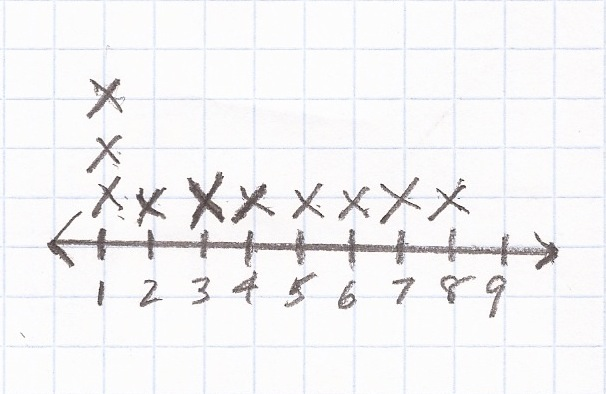## Median

Median = Middle

Basics
Median = Middle
Median Number = Middle Number

The median is the number in the middle of a group of numbers.
Half of the numbers are higher than the median; half of the numbers are lower than the median.

In order to determine the median, it is helpful to create a row or a column of numbers from lowest to highest.
When there is an odd number of numbers, the median can by identified without calculation.
When there is an even number of numbers, the median must be calculated.

The formula provided for median by the Mathematics Formula Sheet is not presented as a mathematical expression.

Formulas (provided by Mathematics Formula Sheet)
Median is the middle value in an odd number of ordered values of a data set, or the mean of the two middle values in an even number of ordered values in a data set.

Formulas (NOT provided by Mathematics Formula Sheet)
Median for odd number of numbers = Middle Number

Median for even number of numbers =$\bf\displaystyle\frac{Sum\,of\,Two\,Middle\,Numbers}{2}$

Question
What is the median of 1, 2, 3, 4, 5, 6, 7 (an odd number of numbers)?

4

RowColumn
7
6
5
4  <— Median for odd number of numbers = Middle Number
3
2
1

Question
What is the median of 1, 2, 3, 4, 5, 6, 7, 8 (an even number of numbers) in decimal form?

4.5

RowColumn
8
7
6
5
<— 4.5 <— Median for even number of numbers =$\bf\displaystyle\frac{Sum\,of\,Two\,Middle\,Numbers}{2}$
4
3
2
1

Calculator Click What You See Commentblinker clears screen4 + 5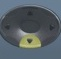2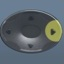$\bf\displaystyle\frac{4+5}{2}$$\bf\displaystyle\frac{Sum\,of\,Two\,Middle\,Numbers}{2}$$\bf\displaystyle\frac{9}{2}$4.5 Answer
(Toggle)
###### Median

Question
Five people weigh 111, 135, 156, 175, and 199 pounds, respectively.
What is their median weight?

156

199
175
156  <— Median for odd number of numbers = Middle Number
135
111

Question

Six people weight 105, 111, 135, 156, 175, and 199 pounds, respectively.
What is their median weight in decimal form?

145.5

199
175
156
<— 145.5 <— Median for even number of numbers =$\bf\displaystyle\frac{Sum\,of\,Two\,Middle\,Numbers}{2}$
135
111
105

Calculator Click What You See Commentblinker clears screen135 + 1562$\bf\displaystyle\frac{135+156}{2}$$\bf\displaystyle\frac{Sum\,of\,Two\,Middle\,Numbers}{2}$$\bf\displaystyle\frac{291}{2}$145.5 Answer
(Toggle)
###### Median

Question
The median weight of five people is 177 pounds.  As per the column below, four of their five weights are known.  Place an X over the question mark where the fifth weight should go.

?
179
177  <— Median
135
111
?

X
179
177  <— Median
135
111

X
179
177  <— Median for odd number of numbers = Middle Number
135
111

Question

This graph is meant to show the age (in years) of nine children.
Place an X on the graph such that the median age is 5.Median for odd number of numbers = Middle Number

The original graph shows the ages of eight children.  Because the final number of children is an odd number, the ninth X must be placed at the number 5 to allow half of the ages to be greater than 5 and half less than 5.

Question

This graph is meant to show the age (in years) of nine children.
Place an X on the graph where the median age should be.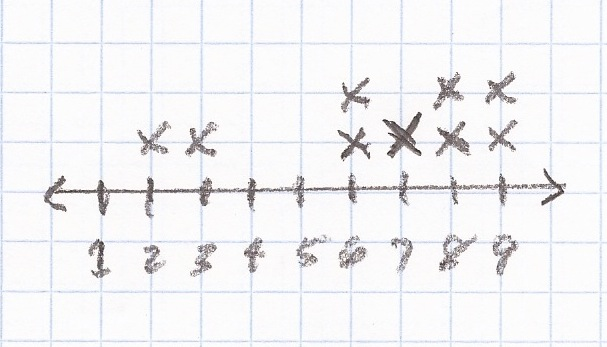Median for odd number of numbers = Middle Number

The original graph shows the ages of eight children.  Because the final number of children is an odd number, the ninth X must be placed at the number 7 to allow half of the ages to be greater than 7 and half less than 7.

Question

This graph is meant to show the age (in years) of ten children.  The median age of the ten children is 5.5.  Place the tenth X on the graph where it should be.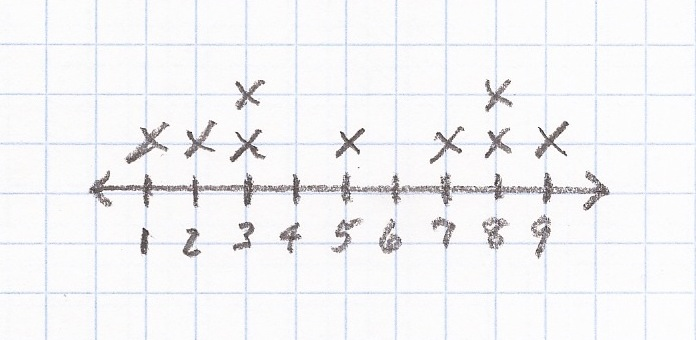The original graph shows the ages of nine children.  Because the given median of 5.5 is halfway between 5 and 6 and there is already an X at the number 5, the tenth X must be placed at the number 6.

Practice – Questions

1.  Three houses cost $125,000,$179,000, and $225,000, respectively. What is their median cost? Show your work. 2. Four houses cost$125,000, $179,000,$225,000, and $276,000, respectively. What is their median cost? Show your work. 3. Five basketball players scored 7, 12, 15, 28, and 33 points, respectively. What was their median score? 4. Six bowlers scored 117, 129, 155, 168, 202, and 233, respectively. What was their median score in decimal form? 5. The median score of seven bowlers is 142. As per the column below, six of their seven scores are known. Place an X over the question mark where the seventh score should go. 245 ? 179 142 <— Median ? 139 135 111 6. This graph is meant to show the age (in years) of nine children. Place an X on the graph such that the median age is 5.7. This graph is meant to show the age (in years) of nine children. Place an X on the graph where the median age should be.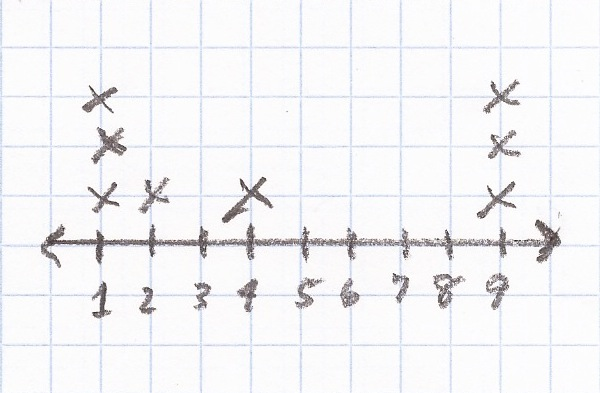8. This graph is meant to show the age (in years) of nine children. Place an X on the graph where the median age should be.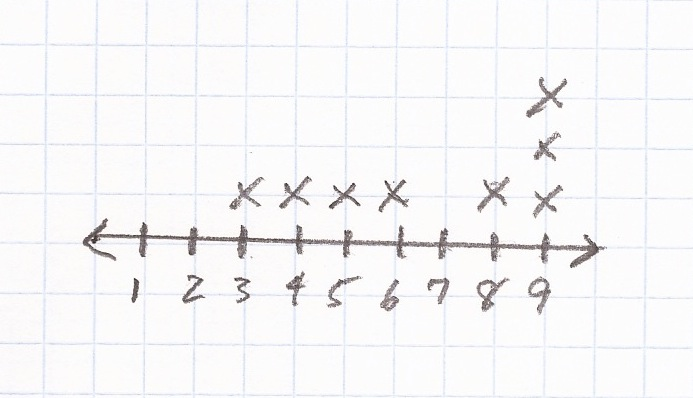9. This graph is meant to show the age (in years) of ten children. The median age of the ten children is 7.5. Place the tenth X on the graph where it should be.10. This graph is meant to show the age (in years) of ten children. The median age of the ten children is 3.5. Place the tenth X on the graph where it should be.Practice – Answers 1.$179,000

$225,000$179,000  <— Median for odd number of numbers = Middle Number
$125,000 2.$202,000

$276,000$225,000
<– $202,000 <– Median for even number of numbers =$\bf\displaystyle\frac{Sum\,of\,Two\,Middle\,Numbers}{2}$$179,000
\$125,000

Calculator Click What You See Commentblinker clears screen179000 + 2250002$\bf\displaystyle\frac{179000+225000}{2}$$\bf\displaystyle\frac{Sum\,of\,Two\,Middle\,Numbers}{2}$202000 Answer
###### Median

3.  15

4.  161.5

5.

245
X
179
142  <— Median for odd number of numbers = Middle Number
139
135
111

6.7.8.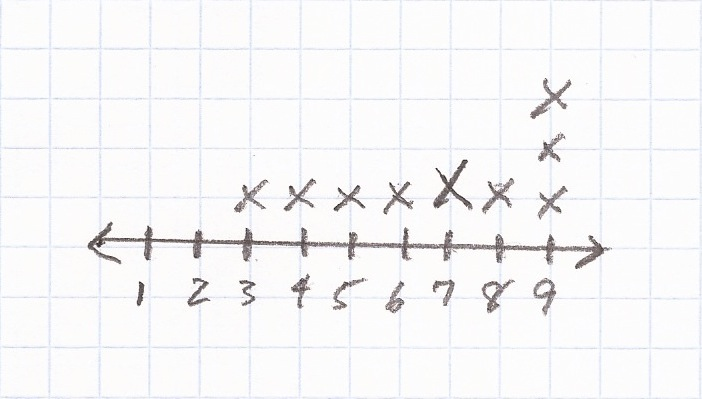10.